﻿ 规则连接神经网络的同步分析与模拟

# 规则连接神经网络的同步分析与模拟Synchronization Analysis and Simulation on Regular Coupled Neural Networks

Abstract: We take Hindmarsh-Rose (HR) model as an example to study the synchronization property of reg-ular coupled neural networks. Through introducing appropriate error variable, we transform the stability of synchronization manifold into that of null solution of error equations. By constructing a proper Lyapunov function for error equations, the relative criterion is theoretically provided for global asymptotic synchronization of HR neural networks. Further, the theoretical result shows the influence of neuron numbers and connection types in neural network on synchronization. Numerical simulations test the reliability of the results in this paper.

1. 引言

2. 模型描述

2.1. 单个HR模型

HR模型是Hindmarsh和Rose根据电压钳实验获得的关于蜗牛神经细胞数据而提出的，它能模拟软体动物神经元重复的峰放电和不规则的簇放电行为，是一类可兴奋的神经元模型。其简化的神经元模型有利于模拟真实神经网络，单个HR模型描述如下：

$\left\{\begin{array}{c}\frac{\text{d}{x}_{1}}{\text{d}t}={x}_{2}+f\left({x}_{1}\right)-{x}_{3}+I,\\ \frac{\text{d}{x}_{2}}{\text{d}t}=g\left({x}_{1}\right)-{x}_{2},\\ \frac{\text{d}{x}_{3}}{\text{d}t}=\mu \left[s\left({x}_{1}-\chi \right)-{x}_{3}\right],\end{array}$ (1)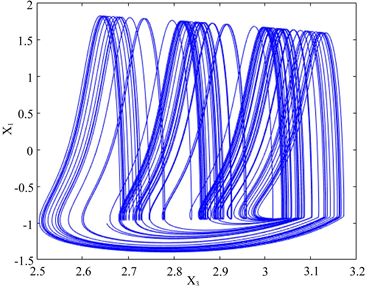(a)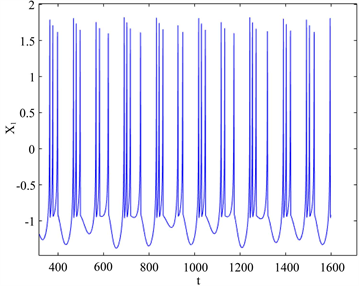(b)

Figure 1. (a) Time series of membrane potential; (b) Phase-plane diagram of x1 and x3

2.2. N个神经元电耦合的动力系统

$\left\{\begin{array}{ll}\frac{\text{d}{x}_{i1}}{\text{d}t}={f}_{1}\left({X}_{i}\right)+\underset{j=1}{\overset{\text{N}}{\sum }}{k}_{ij}{g}_{ij}{x}_{j1},\hfill & \hfill \\ \frac{\text{d}{x}_{i2}}{\text{d}t}={f}_{2}\left({X}_{i}\right),\hfill & \hfill \\ \frac{\text{d}{x}_{i3}}{\text{d}t}={f}_{3}\left({X}_{i}\right),\hfill & i=1,2,3,\cdots ,\text{N,}\hfill \end{array}$ (2)

$\frac{\text{d}{X}_{i}}{\text{d}t}=F\left({X}_{i}\right)+k\underset{j=1}{\overset{N}{\sum }}{g}_{ij}T{X}_{j},i=1,2,3,\cdots ,N,$ (3)

${X}_{1}\left(t\right)={X}_{2}\left(t\right)=\cdots ={X}_{N}\left(t\right)=s\left(t\right),t\to +\infty ,$ (4)

$\frac{\text{d}\gamma }{\text{d}t}=\left({D}_{X}F\left(s\left(t\right)\right)+k{\lambda }_{m}T\right)\gamma ,m=2,3,\cdots ,N,$ (5)

3. 三种连接方式分析

3.1. 链式连接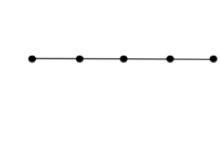(a)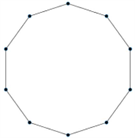(b)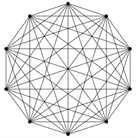(c)

Figure 2. Three different connections: (a) chain connection; (b) ring connection; (c) global connection

$\begin{array}{c}\frac{\text{d}V}{\text{d}t}={\gamma }^{T}\cdot \left({\left[DF\left(s\left(t\right)\right)\right]}^{T}\cdot A+A\cdot DF\left(s\left(t\right)\right)+2k{\lambda }_{m}AT\right)\cdot \gamma \\ ={\gamma }^{T}\cdot \left[\begin{array}{ccc}{f}^{\prime }\left({x}_{1}\right)+k{\lambda }_{m}& \frac{{g}^{\prime }\left({x}_{1}\right)+1}{2}& 0\\ \frac{{g}^{\prime }\left({x}_{1}\right)+1}{2}& -1& 0\\ 0& 0& -\frac{1}{s}\end{array}\right]\cdot \gamma \\ \le \left(H+k{\lambda }_{m}\right){|{\gamma }_{1}|}^{2}+\left(L+1\right)|{\gamma }_{1}{\gamma }_{2}|-{|{\gamma }_{2}|}^{2}-\frac{1}{s}{|{\gamma }_{3}|}^{2}\\ \le \left(|{\gamma }_{1}|,|{\gamma }_{2}|,|{\gamma }_{3}|\right)\cdot \left[\begin{array}{ccc}H+k{\lambda }_{m}& \frac{L+1}{2}& 0\\ \frac{L+1}{2}& -1& 0\\ 0& 0& -\frac{1}{s}\end{array}\right]\cdot {\left(|{\gamma }_{1}|,|{\gamma }_{2}|,|{\gamma }_{3}|\right)}^{T}\end{array}$

$k>\frac{H+\frac{{\left(L+1\right)}^{2}}{4}}{|{\lambda }_{2}|}$ 时， ${B}_{m}=\left[\begin{array}{ccc}H+k{\lambda }_{m}& \frac{L+1}{2}& 0\\ \frac{L+1}{2}& -1& 0\\ 0& 0& -\frac{1}{s}\end{array}\right]<0\left(m=2,3,\cdots ,N\right)$

3.2. 环状连接

3.3. 全局连接

4. 数值模拟

$e\left(t\right)=\sqrt{|\frac{1}{N}\underset{i=1}{\overset{N}{\sum }}{x}_{i1}{\left(t\right)}^{2}-{\left(\frac{1}{N}\underset{i=1}{\overset{N}{\sum }}{x}_{i1}\left(t\right)\right)}^{2}|},$

$e\left(t\right)$ 表示 $t$ 时刻的同步差，它反映了耦合系统的同步状态。规定当耦合强度 $k>{k}_{0}$ 时，如果 $\underset{t\to +\infty }{\mathrm{lim}}e\left(t\right)=0$ ，那么称系统(3)达到了完全同步，此时我们把满足 $k>{k}_{0}$ 条件的 ${k}_{0}$ 的最小值称为耦合系统实际的同步阈值。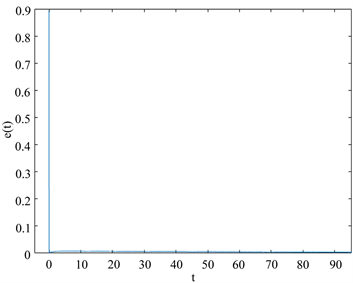(a)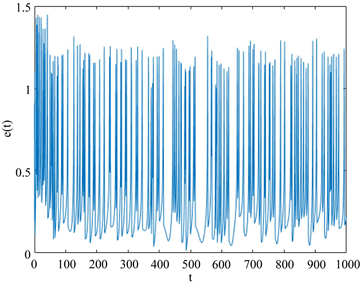(b)

Figure 3. (a) The time series of e(t) when k = 0 in system (3); (b) The time series of e(t) in chain-coupled system when k = 193.3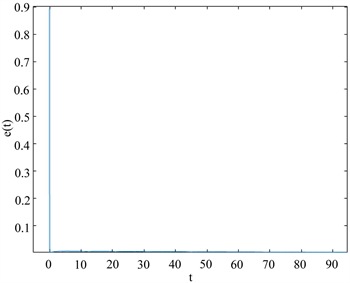(a)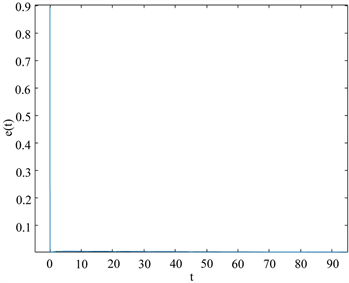(b)

Figure 4. (a) The time series of e(t) in ring-coupled system when k = 56.62; (b) The time series of e(t) in global-coupled system when k = 28.31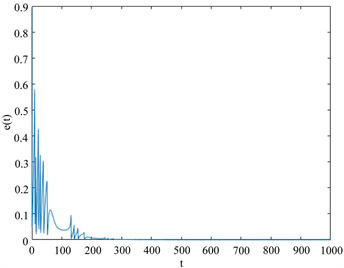(a)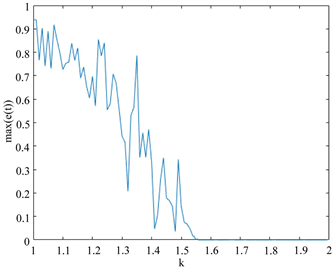(b)

Figure 5. (a) Maximum of e(t) vs. k in chain-coupled system; (b) The time series of e(t) in chain-coupled system when k = 1.56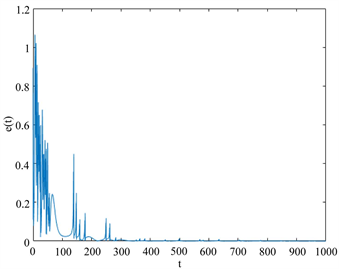(a)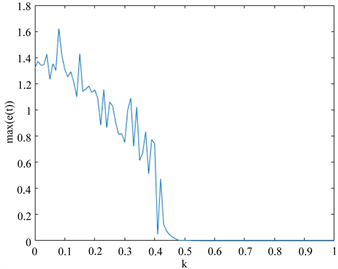(b)

Figure 6. (a) Maximum of e(t) vs. k in ring-coupled system; (b) The time series of e(t) in ring-coupled system when k = 0.47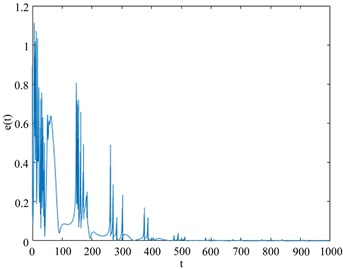(a)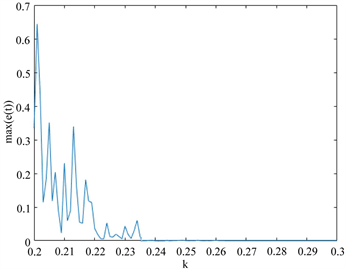(b)

Figure 7. (a) Maximum of e(t) vs. k in global-coupled system; (b) The time series of e(t) in global-coupled system when k = 0.235

$N=5,6,\cdots ,12$ ，重复上述过程。在链式、环状和全局连接方式下，数值模拟得到实际同步阈值分别记作 ${{k}^{\prime }}_{c},{{k}^{\prime }}_{r}$${{k}^{\prime }}_{g}$ 。数据如表1图8表1中神经元数目和同步阈值关系图，它更直观地展示了同步阈值随神经元数目的变化趋势。从表1图8可以看出理论和数值模拟结果都反映出相同的性质，对于链式和环状连接的系统，同步阈值随N的增大而增大。对于全局连接的系统，同步阈值随N的增大而减小。并且理论计算出的同步阈值大约是数值模拟得到的同步阈值的125倍。另外，当N相同的时候，理论计算的同步阈值和数值模拟的同步阈值的关系为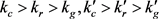，这说明全局连接的系统比环状连接的系统更容易达到同步，而环状连接的系统又比链式连接的系统更容易达到同步。Table 1. Data of the number of neuron and synchronization thresholds for three types of regular coupled neural networks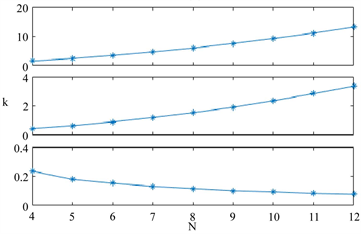(a)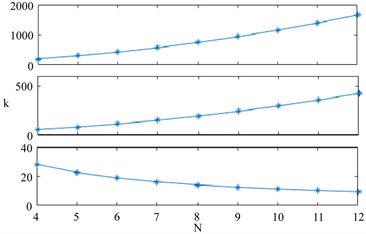(b)

Figure 8. (a) The theoretical synchronization thresholds vs. the number of neurons from top to bottom being chain-coupled, ring-coupled and global-coupled; (b) The true synchronization thresholds vs. the number of neurons from top to bottom being chain-coupled, ring-coupled and global-coupled, respectively

5. 本文结论

 王青云. 神经元耦合系统的同步动力学[M]. 北京: 科学出版社, 2008: 38-51.

 Gray, C.M., k?nig, P., Engel, A.K., et al. (1989) Oscillatory Responses in Cat Visual Cortex Exhibit Inter-Columnar Synchronization Which Reflect Global Stimulus Properties. Nature, 338, 334-337.
https://doi.org/10.1038/338334a0

 Fell, J., Fernandez, G., Klaver, P., et al. (2003) Is Synchronized Neural Gamma Activity Relevant for Selective Attentions. Brain Research Reviews, 42, 265-272.
https://doi.org/10.1016/S0165-0173(03)00178-4

 Mormann, F., Andrzejak, R.K., Kreuz, T., et al. (2003) Automated De-tection of a Preseizure State Based on a Decrease in Synchronization in Intracranial Electroencephalogram Recordings from Epilepsy Patients. Physical Review E, 67, Article ID: 021912.
https://doi.org/10.1103/PhysRevE.67.021912

 Khazipov, R. (2016) GABAergic Synchronization in Epilepsy. Cold Spring Harbor Perspectives in Medicine, 6, Article ID: 022746.
https://doi.org/10.1101/cshperspect.a022764

 Pfurtscheller, G., Pichler-Zalaudek, K., Ortmayr, B., et al. (1998) Post Movement Beta Synchronization in Patients with Parkinson’s Disease. Journal of Clinical Neurophysiology, 15, 243-250.
https://doi.org/10.1097/00004691-199805000-00008

 Hammond, C., Bergman, H. and Brown, P. (2007) Pathological Synchronization in Parkinson’s Disease: Networks, Models and Treatments. Trends in Neurosciences, 30, 360-364.
https://doi.org/10.1016/j.tins.2007.05.004

 Wang, Z. and Zhang, H. (2013) Synchronization Stability in Complex Inter-connected Neural Networks with Nonsymmetric Coupling. Neurocomputing, 108, 84-92.
https://doi.org/10.1016/j.neucom.2012.11.014

 Newman, M.E.J. and Watts, D.J. (1999) Renormalization Group Analysis of the Small-World Network Model. Physics Letters A, 263, 341-346.
https://doi.org/10.1016/S0375-9601(99)00757-4

 Pecora, L.M. and Carroll, T.L. (1998) Master Stability Functions for Synchronized Coupled Systems. Physical Review Letters, 10, 2109-2112.
https://doi.org/10.1103/PhysRevLett.80.2109

 Lu, J.H., Zhou, T.S. and Zhang, S.C. (2002) Chaos Synchronization between Linearly Coupled Chaotic Systems. Chaos Solitons & Fractals, 14, 529-541.
https://doi.org/10.1016/S0960-0779(02)00005-X

 Wang, Q.Y., Lu, Q.S., Chen, G.R., et al. (2006) Chaos Synchronization of Coupled Neurons with Gap Junctions. Physics Letters A, 356, 17-25.
https://doi.org/10.1016/j.physleta.2006.03.017

 赵勇, 吴婵媛, 孟盼. 三个电耦合神经元系统同步分析和模拟[J]. 动力学与控制学报, 2016, 14(3): 269-275.

 Gu, H.G., Li, Y.Y., Jia, B., et al. (2013) Parameter-Dependent Synchronization Transition of Coupled Neurons with Co-Existing Spiking and Bursting. Physica A: Statistical Mechanics and Its Applications, 392, 3281-3292.
https://doi.org/10.1016/j.physa.2013.03.039

 Ren, G., Xu, Y. and Wang, C. (2017) Synchronization Behavior of Coupled Neuron Circuits Composed of Memristors. Nonlinear Dynamics, 88, 893-901.
https://doi.org/10.1007/s11071-016-3283-2

Top